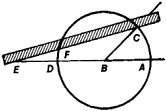# trisection of an angle

## trisection of an angle:

see geometric problems of antiquitygeometric problems of antiquity,
three famous problems involving elementary geometric constructions with straight edge and compass, conjectured by the ancient Greeks to be impossible but not proved to be so until modern times.
.
The following article is from The Great Soviet Encyclopedia (1979). It might be outdated or ideologically biased.

## Trisection of an Angle

the problem of dividing an arbitrary angle into three equal parts. Along with the two other classic problems of ancient Greek mathematics—the squaring, or quadrature, of the circle and the duplication of the cube—the problem of the trisection of an angle played an important role in the development of mathematical methods.

Initially, attempts were made to solve the problem through the use of just the simplest geometric instruments, that is, a straightedge and compasses. Such a solution proved possible only in a few special cases—for example, for angles of 90° and 9072n, where n is a natural number. In the general case, an angle cannot be trisected by means of only a straightedge and compasses. A rigorous proof of this fact (that is, a proof that a solution involving square roots cannot be found for the cubic equation to which the trisection problem reduces) was not given until the 19th century.Figure 1

The problem can be solved if we are not limited to a straight edge and compasses. Archimedes (third century B.C.), for exam ple, set forth a method that makes use of compasses and a straightedge with markings. In order to trisect the angle ABC (see Figure 1) by his method, the distance EF = BA is marked off on the straightedge. The straightedge is adjusted so that it passes through the point C, E falls on the continuation of the diameter AD, and F falls on the periphery of the circle. The angle AEF is then equal to one-third of the angle ABC.